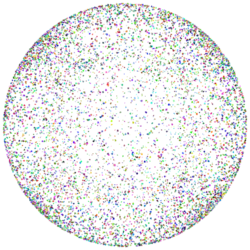### RenderMan Procedural PrimitivesRiPoints on a Sphere

 This tutorial follows on from the tutorial "Procedural Primitives: Basics". Procedural primitives, or helper apps, are ideal when complex surfaces can be defined procedurally. A procedural primitive can be loosely described as a shape made from geometry that has been assembled according to the application of one or more rules. This tutorial introduces a simple procedural primitive made from light-weight ie. fast to render, RenderMan points. The spherical shell shown in figure 1 consists of 5000 points. Using a Intel MacOSX 667 MHz the following timings were obtained,     1,000,000 points generated in 1 min 12 seconds     100,000 points generated in 7 seconds     10,000 points generated in 0 seconds !Figure 1 10,000 random points generated by ripoint.pyFigure 2 10,000 random points generated by ripoint.tcl

 The rib statement that requests the renderer to produce, say, three points of uniform radius, colored red, green and blue is, ``` Points "P" [-0.5 0 0 0 0 0 0.5 0 0] "constantwidth" [0.01] "Cs" [1 0 0 0 1 0 0 0 1]``` There can be any number of xyz's following the "`P`" parameter, each triplet specifies the position of a point. If the points are to have a specific color there must be as many rgb color values following the "`Cs`" parameter as there are xyz's in the "`P`" list.

#### Spherical Shell of Points

The method for producing a spherical shell of randomly placed colored points is,

1. generate a random vector in a unit cube
2. normalize the vector
3. scale the vector by "radius"
4. use the vectors components to locate a point in space
5. generate a random color

By repeating these steps we obtain a spherical shell of points. The cloud proc uses three functions that were developed in other tutorials,

#### Rib File for Testing

Listing 1 is a rib file that can be used to test both the python and the Tcl implementations of the helper app. The rib file is setup for use on MacOSX.

Listing 1 (ripoints.rib)

 ```#Option "statistics" "endofframe"  Display "shader_tester" "it" "rgba" Format 250 250 1 Projection "perspective" "fov" 40 ShadingRate 1 Translate 0 0 6 Rotate 0 1 0 0 Rotate 0 0 1 0 Scale 1 1 -1 WorldBegin AttributeBegin Surface "constant" Procedural "RunProgram" ["/usr/bin/python FULL_PATH/ripoints.py" "2 10000 0.02"] [-2 2 -2 2 -2 2] #Procedural "RunProgram" ["/usr/bin/tclsh FULL_PATH/ripoints.tcl" "2 10000 0.02"] # [-2 2 -2 2 -2 2] AttributeEnd WorldEnd ```

#### Python Implementation

Listing 2 (ripoints.py)

 ```import sys, math, random random.seed(5) def randBetween(min, max): return random.random() * (max - min) + min def length(x, y, z): return math.sqrt(x*x + y*y + z*z) def normalize(x, y, z): len = length(x, y, z) return x/len, y/len, z/len def scaleVector(x, y, z, sc): return x*sc, y*sc, z*sc def cloud(radius, num, width): print 'Points \"P\" [' for n in range(num): x = random.random() * 2 - 1; y = random.random() * 2 - 1; z = random.random() * 2 - 1; x,y,z = normalize(x, y, z) x,y,z = scaleVector(x, y, z, radius) print '%s %s %s' % (x, y, z) print '] \"constantwidth\" [%s]' % width print '\"Cs\" [' for n in range(num): r = randBetween(0, 1) g = randBetween(0, 1) b = randBetween(0, 1) print '%s %s %s' % (r, g, b) print ']' def main(): args = sys.stdin.readline() while args: arg = args.split() pixels = float(arg) rad = float(arg) num = int(arg) width = float(arg) print 'TransformBegin' cloud(rad, num, width) print 'TransformEnd' sys.stdout.write('\377') sys.stdout.flush() # read the next set of inputs args = sys.stdin.readline() if __name__ == "__main__": main() ```

#### Tcl Implementation

Listing 3 (ripoints.tcl)

 ```fconfigure stdout -translation binary proc randBetween { min max } { return [expr rand() * (\$max - \$min) + \$min] } proc length { x y z } { return [expr sqrt(\$x*\$x + \$y*\$y + \$z*\$z)] } proc normalize { x y z } { set len [length \$x \$y \$z] return [list [expr \$x/\$len] [expr \$y/\$len] [expr \$z/\$len]] } proc scaleVector { vect sc } { set X [expr [lindex \$vect 0] * \$sc] set Y [expr [lindex \$vect 1] * \$sc] set Z [expr [lindex \$vect 2] * \$sc] return [list \$X \$Y \$Z] } proc cloud { radius num width } { puts "Points \"P\" \[" for {set n 0} {\$n < \$num} {incr n} { set x [expr rand() * 2 - 1] set y [expr rand() * 2 - 1] set z [expr rand() * 2 - 1] set vec [normalize \$x \$y \$z] set vec [scaleVector \$vec \$radius] puts "[lindex \$vec 0] [lindex \$vec 1] [lindex \$vec 2] " } puts "\] \"constantwidth\" \[\$width\]" puts "\"Cs\" \[" for {set n 0} {\$n < \$num} {incr n} { set r [randBetween 0 1] set g [randBetween 0 1] set b [randBetween 0 1] puts "\$r \$g \$b " } puts "\]" } while { [gets stdin args] != -1 } { set pixels [lindex \$args 0] set rad [lindex \$args 1] set num [lindex \$args 2] set width [lindex \$args 3] puts "AttributeBegin" cloud \$rad \$num \$width puts "AttributeEnd" puts "\377" flush stdout }```

#### Animation

If the radius of the cloud is increased over several frames it would create the illusion of a fireworks explosion. The ripoints scripts implemented in this tutorial could be instanced by each particle in a Maya or Houdini particle system. When viewed within the modeler, such a particle system might look relatively unimpressive, however, the final particle system would appear to be complex.